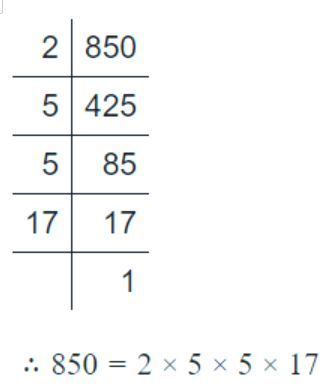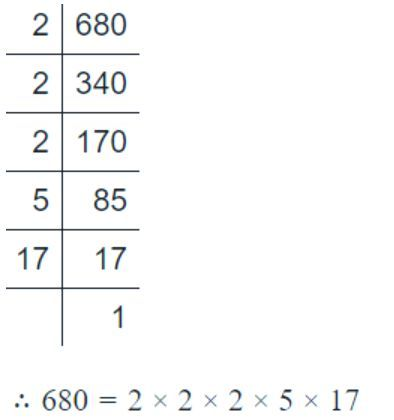Home/Class 6/Maths/

## QuestionMathsClass 6

Two  tankers contain 850 litres and 680 liters of kerosene oil Respectively.  Find the maximum capacity of a container which can measure the kerosene oil  of both the tankers when used an exact number of times.

170 liters.
4.64.6## Solution

H.C.F.  of (850,380)Therefore H.C.F. of (850,680) = $$2\times 5\times 17=170$$
The maximum capacity of a container,which can measure the kerosene oil  of both the tankers when used an exact number of times is170 liters.